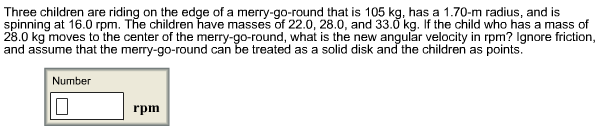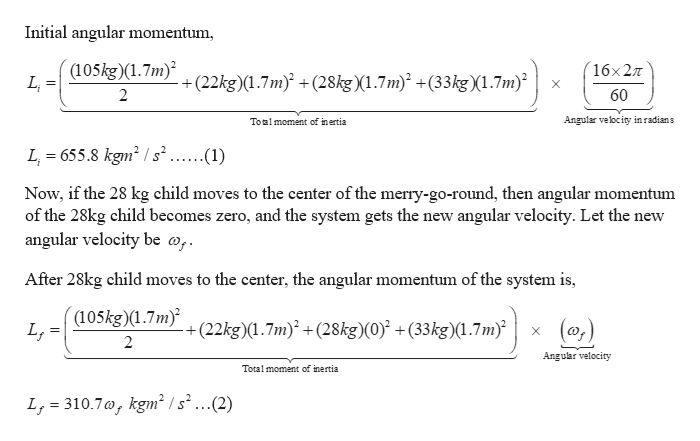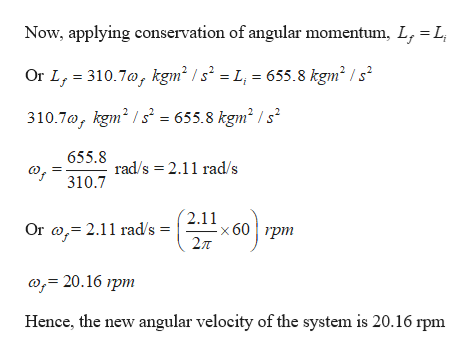# Three children are riding on the edge of a merry-go-round that is 105 kg, has a 1.70-m radius, and isspinning at 16.0 rpm. The children have masses of 22.0, 28.0, and 33.0 kg. If the child who has a mass of28.0 kg moves to the center of the merry-go-round, what is the new angular velocity in rpm? Ignore frictionand assume that the merry-go-round can be treated as a solid disk and the children as points.Numberrpm

Questionhelp_outlineImage TranscriptioncloseThree children are riding on the edge of a merry-go-round that is 105 kg, has a 1.70-m radius, and is spinning at 16.0 rpm. The children have masses of 22.0, 28.0, and 33.0 kg. If the child who has a mass of 28.0 kg moves to the center of the merry-go-round, what is the new angular velocity in rpm? Ignore friction and assume that the merry-go-round can be treated as a solid disk and the children as points. Number rpm fullscreen
check_circleExpert Solution
Step 1

Given:

Mass of the merry-go-round, M = 105 kg

Radius of the merry-go-round = 1.7 m

Angular velocity = 16 rpm

Mass of the 1st child = 22 kg

Mass of 2nd child = 28 kg

Mass of 3rd child = 33 kg

Merry-go-round can be treated as solid disk and children as points.

Step 2

Calculating the new angular velocity of the merry-go-round if the 28 kg child moves to the center:

As there is no external force involved in the merry-go-round, so the total angular momentum of the system (merry-go-round plus children) will remain conserved.help_outlineImage TranscriptioncloseInitial angular momentum (105kg)(1.7m) LL 16*27T +(22kg)(1.7m +(28kg)(1.7m) +(33kg)(1.7m) X 2 60 Angular velocity inradians Toalmoment of inertia L 655.8 kgm2 /s ......(1) Now, if the 28 kg child moves to the center of the merry-go-round, then angular momentum of the 28kg child becomes zero, and the system gets the new angular velocity. Let the new angular velocity be a, After 28kg child moves to the center, the angular momentum of the system is, (105kg)(1.7m LT +(22kg)(1.7m) +(28kg)(0) +(33kg)(1.7mx (0, 2 Angular velocity Total moment of inertia L, 310.7a, kgm2 /s2...(2) fullscreen
Step 3

Now applying the conservation of angular momentum to calculate the f...help_outlineImage TranscriptioncloseL Now, applying conservation of angular momentum, L, Or L, 310.7a, kgm2 /s2 = L, = 655.8 kgm2 / s2 310.7a, kgm2s= 655.8 kgm2 /s2 655.8 о, rad/s 2.11 rad/s 310.7 2.11 x 60 pm 2л ( 2.11 rad/s = Or a; 20.16 rpm Hence, the new angular velocity of the system is 20.16 rpm fullscreen

### Want to see the full answer?

See Solution

#### Want to see this answer and more?

Solutions are written by subject experts who are available 24/7. Questions are typically answered within 1 hour*

See Solution
*Response times may vary by subject and question
Tagged in

### Angular Motion